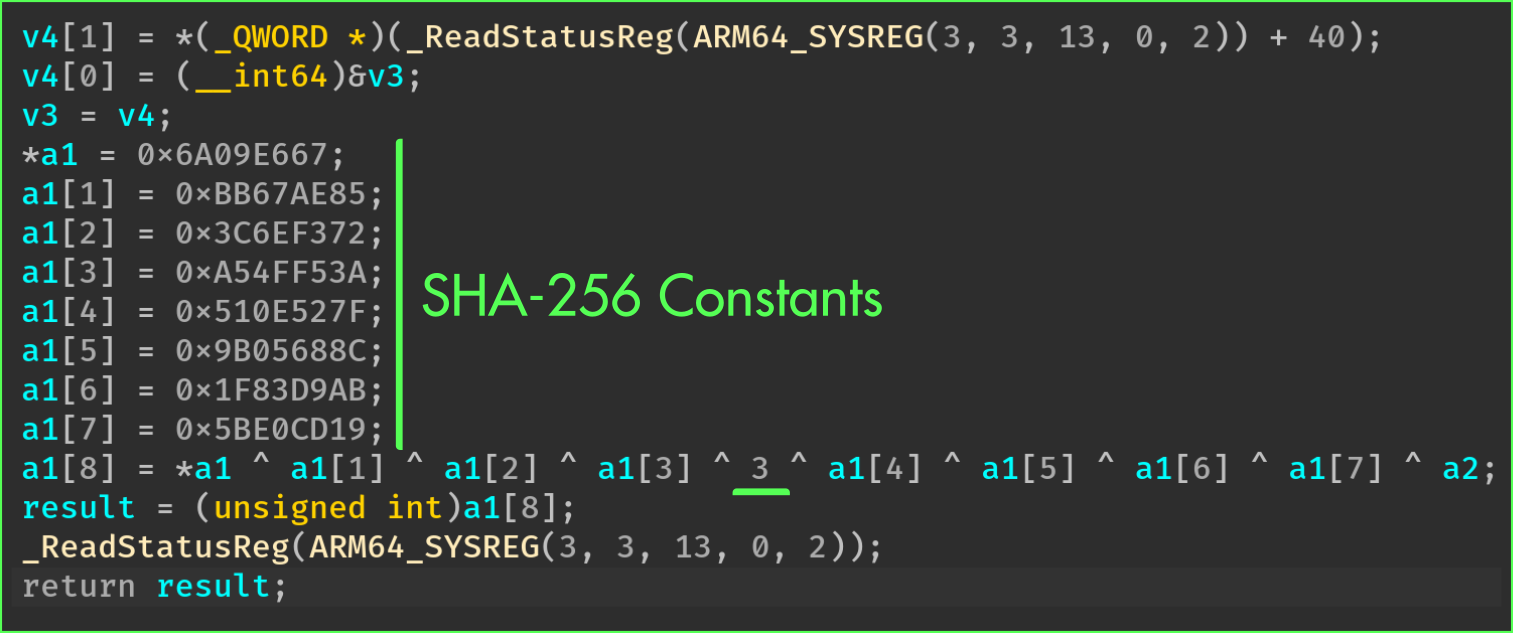# Opaque Constants

While reverse engineering binary, clear strings, symbols, and integer constants are important information that is used to quickly infer the purpose of a function.

This pass protects integer constants by using opaque operations.

## When to use it?

You should use it if your function is using integer constants that characterizes its logic. This is particularly true for cryptographic and encoding functions.

You could also trigger this pass to add another layer of protection on a sensitive function, even though the constants of the function are not sensitive.

## How to use it?

As for the other passes, this protection can be enabled by defining the function `obfuscate_constants` in the class that inherits from `omvll.ObfuscationConfig`:

``````class Config(omvll.ObfuscationConfig):
def obfuscate_constants(self, mod: omvll.Module, func: omvll.Function):
# Logic goes here
``````

The returned values of this method depend on which constants should be protected. Let’s take a real example with the SHA-256 initialization routine. Usually, this routine initializes a buffer with the SHA-256 constants. For instance, in mbedtls it is implemented as follows:

``````int mbedtls_sha256_starts(mbedtls_sha256_context *ctx, int is224) {
if (!is224) {
/* SHA-256 */
ctx->state = 0x6A09E667;
ctx->state = 0xBB67AE85;
ctx->state = 0x3C6EF372;
ctx->state = 0xA54FF53A;
ctx->state = 0x510E527F;
ctx->state = 0x9B05688C;
ctx->state = 0x1F83D9AB;
ctx->state = 0x5BE0CD19;
}
}
``````

For the purpose of this documentation, let’s slightly modify this routine:

``````uint32_t init_context_all(context_t& ctx, uint32_t secret) {
ctx.state = 0x6A09E667;
ctx.state = 0xBB67AE85;
ctx.state = 0x3C6EF372;
ctx.state = 0xA54FF53A;
ctx.state = 0x510E527F;
ctx.state = 0x9B05688C;
ctx.state = 0x1F83D9AB;
ctx.state = 0x5BE0CD19;
ctx.result = ctx.state ^ ctx.state ^ ctx.state ^ ctx.state ^ 0x03 ^
ctx.state ^ ctx.state ^ ctx.state ^ ctx.state ^ secret;
return ctx.result;
}
``````

Once compiled, this function looks like this in a decompiler:From this output, we can clearly identify the initialization constants.

To protect all the constants, we can return `True` from the configuration callback:

``````class Config(omvll.ObfuscationConfig):
def obfuscate_constants(_, __, func: omvll.Function):
if "init_context_all" in func.demangled_name:
return True
return False
``````

And `False` for none of them. As a result, here are the differences before and after enabling the pass:

We might also want to only protect a subset of constants. In that case, we can return a lower limit for which only the constants above this limit will be obfuscated:

``````class Config(omvll.ObfuscationConfig):
def obfuscate_constants(_, __, func: omvll.Function):
if "init_context_all" in func.demangled_name:
# Obfuscate the constants that are greater (strict) than 3
return omvll.OpaqueConstantsLowerLimit(3)
return False
``````

With such a configuration, the constants below or equal to 3 are not obfuscated:

Finally, the function can also return a list of constants that must be obfuscated:

``````class Config(omvll.ObfuscationConfig):
def obfuscate_constants(_, __, func: omvll.Function):
if "init_context_all" in func.demangled_name:
# Only obfuscate the constants that are in this list
return [0x510E527F, 0x9B05688C, 0x1F83D9AB, 0x5BE0CD19]
return False
``````

## Implementation

This pass works by iterating over all the instruction’s operands and by checking if it is a constant:

``````for (Function& F : M) {
for (BasicBlock& BB : F) {
for (Instruction& I : BB) {
for (Use& Op : I.operands()) {
if (auto* CI = dyn_cast<ConstantInt>(Op)) {
Process(I, Op, *CI, opt);
} } } } }
``````

Upon a constant, the pass generates the same constant under an opaque form:

###### Opaque Zero

The pass generates an opaque zero value as follows:

``````0 == MBA(X ^ Y)        - (X ^ Y)
0 == (X | Y) - (X & Y) - (X ^ Y)
``````
###### Opaque One

Opaque 1 leverages the fact that the stack is aligned, which means that the lower bits are set to 0:

``````LSB = LSB(stack address)
Odd = random_odd()

1 == (LSB + Odd) % 2
``````
###### Opaque Value

To obfuscate a random constant that is not 0 or 1, the pass randomly splits the constant in two parts:

``````uint64_t Val = CST; // <--- To protect

uint8_t Split = random(1, min(255, Val));

uint64_t LHS = Val - Split; // Part 1
uint64_t RHS = Split;       // Part 2
``````

Then, the pass generates IR instructions to reconstruct the original value. This reconstruction is essentially an addition between `LHS` and `RHS` and to prevent a “constant propagation”, it uses two intermediate variables:

1. `__omvll_opaque_gv`
2. `__omvll_opaque_stack_allocated`

The pass also adds an opaque zero using the lower bits of the stack address. In the end, the transformation of the constant looks like this:

``````static uint64_t __omvll_opaque_gv;

void function() {
uint64_t __omvll_opaque_stack_allocated;
__omvll_opaque_gv              = LHS;
__omvll_opaque_stack_allocated = RHS;

__omvll_opaque_gv              += OpaqueZero(0);
__omvll_opaque_stack_allocated += OpaqueZero(0);

// The original value:
uint64_t Val = __omvll_opaque_gv + __omvll_opaque_stack_allocated;
}
``````

## Limitations

In its current form, the opaque values are generated with the same transformation. Hence, if an attacker manages to automate the deobfuscation, the attack scales easily.

If the reverse engineering tools (IDA, BinaryNinja, …) introduce assumption about lower bits of the stack, it could also threaten the pass.